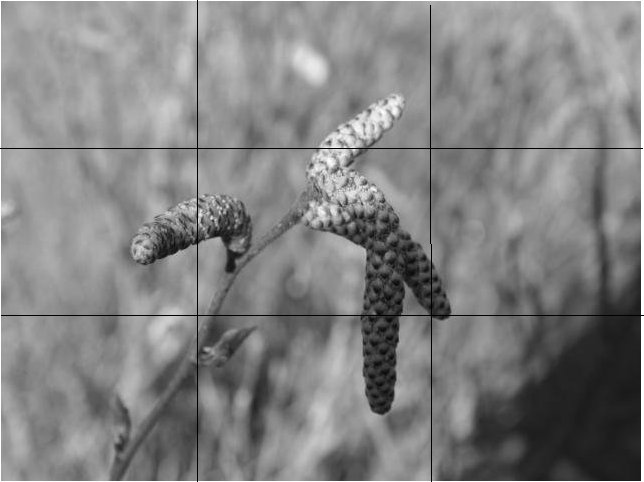## Image Blur Detection via Hough Transform — III

I will continue where I left off in my previous post. After performing Hough transform, and extracted the longest sections of lines for each corresponding Hough line detected, we will need to calculate the gradients of the image pixels luminance around the line sections.

If you remember how the Hough parameters were determined (in polar form, see figure below), it is not difficult to obtain the pixel coordinates centered around the points on the detected line.

In fact, we can formulate a line that is perpendicular to the line section detected during Hough transform, and use the line section that is within a predefined region (e.g. the between the dotted lines) to calculate the gradients of luminance. The following code snippet shows how the perpendicular line’s parameters are obtained.

```    /**
* Get the equation parameters for the line that passes through (x,y) and is perpendicular
* to the line specified by parameters (p0, theta0) in normal form.
*
* @param p0 : distance to line from origin.
* @param theta0 : the slope of p0.
* @param x : x coordinate of the point where the perpendicular line passes through
* @param y : y coordinate of the point where the perpendicular line passes through
* @param &p : the perpendicular line's distance from origin.
* @param &theta : the slope of p.
**/
void LineUtils::GetPerpendicularLineParameters(float p0, float theta0, float x, float y, float &p, float &theta) {
float x0 = p0 * cos(theta0);
float y0 = p0 * sin(theta0);

p = sqrt((x0 - x)*(x0 - x) + (y0 - y)*(y0 - y));

float a1 = theta0 - PI / 2.0;
float a2 = theta0 + PI / 2.0;

//d=|x0 * cos a + y0 * sin a - p|
float d1 = abs(x * cos(a1) + y * sin(a1) - p);
float d2 = abs(x * cos(a2) + y * sin(a2) - p);

if (d1 < d2)
theta = a1;
else
theta = a2;
}
```

In an ideal situation, the gradient of the line points obtained via the method above looks like the figures below, where the edges are clearly identified.

Sometimes, when the lighting condition is poor, the image would appear to be “grainy”, which sometimes led to poor line detection. For instance, the following figure shows the the gradient when the region around the detected edge is grainy:

We use the number of times the luminance increases above or decreases below its mean along the perpendicular line interval as a measure of whether we accept the detection results as gradients or not. Typically when such number of crossings is less than 3 (see the first two images above) the curve is either monotonic or has a single peak, we assume that gradients can be correctly calculated and if the crossings are more than 3 we discard the results. The gradient is calculated as the slope of the curve. In the examples above, we used ten pixels on each side of the Hough line to calculate gradients.

### Image Regions

For images with complex contents, it becomes difficult for the Hough transform to reliably identify line structures within the image. Future more, certain photography techniques (i.e. Bokeh) leave portions of images deliberately blurred. Without dividing image into different sub-regions, the classification results would be compromised.

Thus, images are divided into 9 (3×3) sub-images after Canny edge detection, and Hough transform is performed against each sub-images. The figure below illustrates how an image is divided:Image divided into 3x3 sub-images (Microsoft Research Digital Image)

This technique is especially useful when portions of images are deliberately blurred, like the image shown above. It also helps the line detection accuracy when the image contains complex scenes. By dividing up the image, each sub area’s complexity is greatly reduced. Other methods in scene separation might achieve even better results, but it is out of the scope for our discussion here.

In my next post, I will show some results obtained from using the method mentioned in this and the previous articles and will also discuss its limitations.

1.Marc says:
2.Abhay Kumar Singh says: